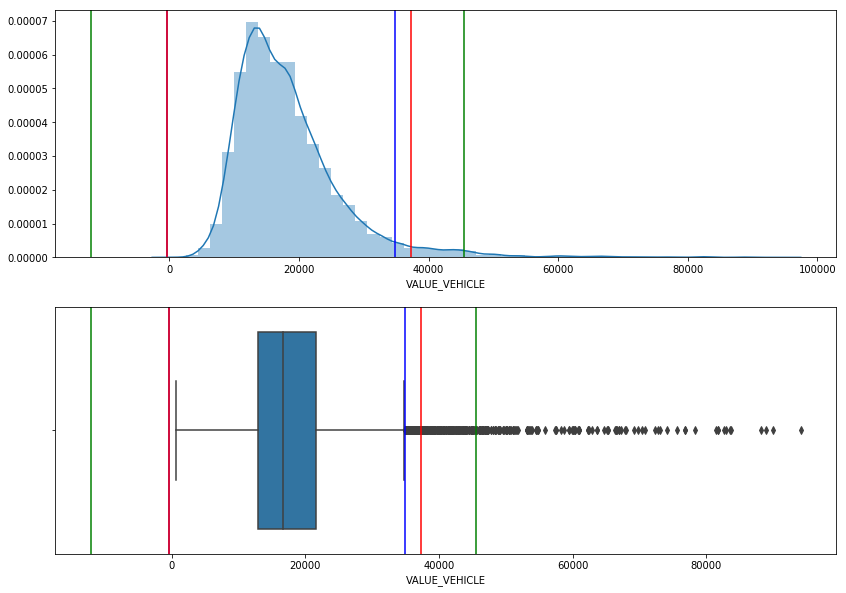Using Statistics to Deal With Invalid Values

Last updated: May 24th, 2019Using statistics to deal with invalid values¶

On previous lectures we saw how to check our data domain and remove values that don't fit our validity domain.

Although our data could be within a valid domain, we might need to see how it is distributed and find deviations markedly from other observations.Hands on!¶

In :
import numpy as np
import pandas as pd
import matplotlib.pyplot as plt
import seaborn as sns

%matplotlib inline


We'll use the data/windscreen.csv file that contains fictitious data for the year 2011 of a car insurance company. Specifically, it is a table where each observation corresponds to an insured person, where you have information specific to the insured (age, city, type of car, etc.), the total cost for relative claims to the moons of your car (INCURR_COST), the number of accidents of this type that has suffered (N_CLAIMS) and its exposure (EXPOSURE), that is, the fraction of year that the subject has been insured.

In :
df = pd.read_csv('data/windscreen.csv', sep=';')


Out:
YEAR EARNED_PREMIUM INSTALLMENT GENDER AGE_DRIVER AGE_LICENSE AGE_ADD_DRIVER AGE_VEHICLE CITIES BRAND_TEXT ... VALUE_VEHICLE POSTCODE N_CLAIMS INCURR_COST EXPOSURE ANTPOL HPOWERPOT DES_ZONE2 DES_ZONE FUEL2
0 2011 15.040385 2 1 44 17 9999 10 46 NISSAN ... 14875.05 46019 0 0.0 0.87 1 18.800000 Urbano Capital D
1 2011 16.066171 2 1 24 3 9999 5 50 FORD ... 14910.00 50196 0 0.0 1.00 1 19.333333 Rural Corona D
2 2011 5.740437 1 1 51 18 9999 1 21 FORD ... 20400.00 21560 0 0.0 0.30 1 14.963636 Rural Rural - Tam 2 D
3 2011 21.426893 1 1 59 40 9999 9 46 FORD ... 23170.00 46014 0 0.0 1.00 1 15.744000 Urbano Capital D
4 2011 16.215815 2 1 47 25 9999 4 8 FORD ... 16420.00 8013 0 0.0 1.00 1 16.500000 Urbano Capital D
5 2011 13.709280 1 1 61 42 9999 6 43 FORD ... 17200.00 43791 0 0.0 1.00 1 26.000000 Rural Rural - Tam 2 D
6 2011 0.994614 1 1 54 35 9999 5 8 FORD ... 15800.00 8013 0 0.0 0.04 1 15.666667 Urbano Capital D
7 2011 1.711628 1 1 38 20 9999 10 8 CITROEN ... 17837.00 8820 0 0.0 0.07 1 23.943662 SubUrb Corona D
8 2011 14.812240 1 1 50 14 9999 14 6 SEAT ... 11299.03 6100 0 0.0 0.34 1 16.966667 Rural Corona G
9 2011 38.401618 1 1 33 9 9999 4 23 PEUGEOT ... 27140.00 23440 0 0.0 1.00 1 11.066176 Rural Rural - Tam 4 D

10 rows × 22 columns

In :
df.describe()

Out:
YEAR EARNED_PREMIUM INSTALLMENT GENDER AGE_DRIVER AGE_LICENSE AGE_ADD_DRIVER AGE_VEHICLE CITIES HPOWER VALUE_VEHICLE POSTCODE N_CLAIMS INCURR_COST EXPOSURE ANTPOL HPOWERPOT
count 10065.0 10065.000000 10065.000000 10065.000000 10065.000000 10065.000000 10065.000000 10065.000000 10065.000000 10065.000000 10065.000000 10065.000000 10065.000000 10065.000000 10065.000000 10065.000000 10065.000000
mean 2011.0 22.713096 1.279583 1.229011 51.263686 27.398013 8913.797218 10.456036 24.195926 6.832290 18480.874459 24580.546349 0.040238 10.917549 0.773231 1.738301 13.895295
std 0.0 17.228943 0.486631 0.430034 140.793326 141.084307 3104.556407 4.897791 14.647140 2.082912 9617.431141 14656.190648 0.205427 62.784658 0.320347 0.802587 14.314716
min 2011.0 0.029860 1.000000 1.000000 20.000000 1.000000 18.000000 0.000000 1.000000 1.000000 631.060000 1002.000000 0.000000 -0.010000 0.010000 1.000000 2.110092
25% 2011.0 12.205191 1.000000 1.000000 40.000000 16.000000 9999.000000 7.000000 9.000000 5.000000 12850.000000 9370.000000 0.000000 0.000000 0.530000 1.000000 11.477941
50% 2011.0 19.853687 1.000000 1.000000 49.000000 25.000000 9999.000000 10.000000 24.000000 7.000000 16695.000000 24400.000000 0.000000 0.000000 1.000000 2.000000 13.333333
75% 2011.0 29.711534 2.000000 1.000000 58.000000 35.000000 9999.000000 13.000000 37.000000 8.000000 21665.000000 37004.000000 0.000000 0.000000 1.000000 2.000000 15.588235
max 2011.0 589.804274 9.000000 8.000000 9999.000000 9999.000000 9999.000000 58.000000 52.000000 13.000000 404736.000000 52006.000000 3.000000 1021.420000 1.000000 3.000000 999.000000
In :
plt.figure(figsize=(14,6))
plt.xticks(rotation=90)

sns.boxplot(x='variable', y='value', data=df._get_numeric_data().melt())

Out:
<matplotlib.axes._subplots.AxesSubplot at 0x7f763e570a20>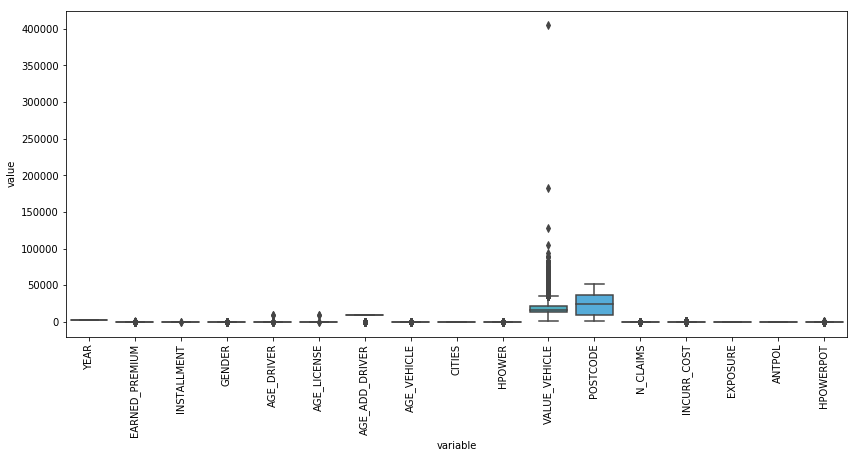VALUE_VEHICLE column¶

Here we have two plots, a density plot and a box plot. This second one is a good way to view the data and, as we can see, there is clearly one data point that seems to be an outlier value.

In :
fig, axs = plt.subplots(2, 1, sharex=True, figsize=(14,10))

sns.distplot(df['VALUE_VEHICLE'], ax=axs)
sns.boxplot(df['VALUE_VEHICLE'], ax=axs)

Out:
<matplotlib.axes._subplots.AxesSubplot at 0x7f763f08ff28>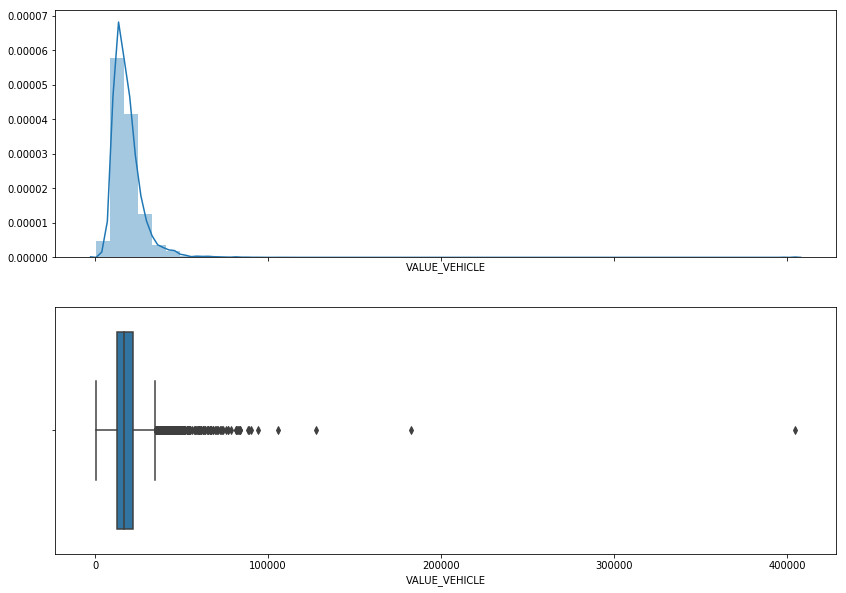In :
df['VALUE_VEHICLE'].describe()

Out:
count     10065.000000
mean      18480.874459
std        9617.431141
min         631.060000
25%       12850.000000
50%       16695.000000
75%       21665.000000
max      404736.000000
Name: VALUE_VEHICLE, dtype: float64

There is a lot of variability, and it seems to be a wrong value at ~400K, lets show the full record:

In :
df.loc[df['VALUE_VEHICLE'] > 400_000, ['VALUE_VEHICLE', 'BRAND_TEXT', 'AGE_VEHICLE']]

Out:
VALUE_VEHICLE BRAND_TEXT AGE_VEHICLE
7779 404736.0 MAYBACH 9

It's a Maybach luxury german car, so this record is correct and we may keep it.EARNED_PREMIUM column¶

This column means the profit obtained with the insurance contract.

We'll show a density plot and a box plot.

In :
fig, axs = plt.subplots(2, 1, sharex=True, figsize=(14,10))


Out:
<matplotlib.axes._subplots.AxesSubplot at 0x7f763efb1f28>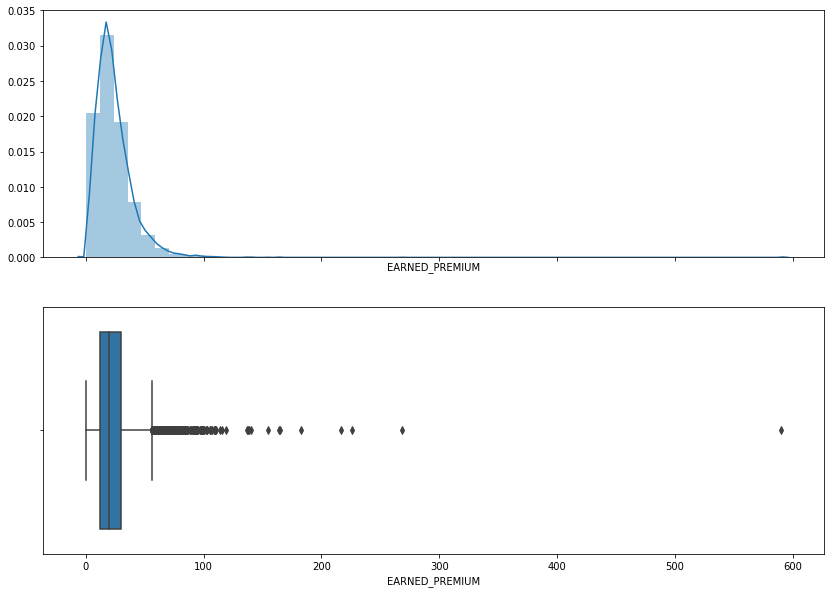In :
df['EARNED_PREMIUM'].describe()

Out:
count    10065.000000
mean        22.713096
std         17.228943
min          0.029860
25%         12.205191
50%         19.853687
75%         29.711534
max        589.804274
Name: EARNED_PREMIUM, dtype: float64

We have a lot of variability and it seems to be a wrong value at ~600, lets show the full record:

In :
df.loc[df['EARNED_PREMIUM'] > 580, ['EARNED_PREMIUM', 'BRAND_TEXT', 'AGE_VEHICLE']]

Out:
7779 589.804274 MAYBACH 9

It's a Maybach luxury german car, so this record is correct and we may keep it. Insurance cost is high, so profit obtained should be high too.VALUE_VEHICLE vs EARNED_PREMIUM¶

The higher VALUE_VEHICLE the higher EARNED_PREMIUM we expect as insurance company:

• High VALUE_VEHICLE with low EARNED_PREMIUM is bad for insurance company.
• Low VALUE_VEHICLE with high EARNED_PREMIUM is good for insurance company.
In :
plt.figure(figsize=(6,6))


Out:
<matplotlib.axes._subplots.AxesSubplot at 0x7f763f4c2048>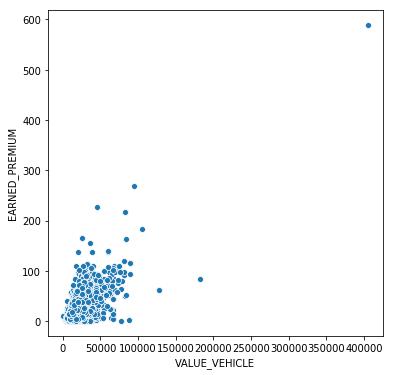Determine the min and max values allowed¶

We determine the min and max cuttoffs for detecting the outliers. There are many ways of doing this:

• Interquartile Range (IQR)
• Mean ± 1.96 Standard deviation: supposing values follow a Normal distribution, we can said that 95% of the values will be within 1.96 standard deviations from the mean.
• Median ± 3 Standard deviation: we defined this approach, as median is a more robust measure of central tendency when the data contains non-balanced data.

We'll go back to the VALUE_VEHICLE column and apply these strategies:

In :
q75, q25 = np.percentile(df['VALUE_VEHICLE'], [75 ,25])
iqr = q75 - q25

min_iqr = q25 - (iqr*1.5)
max_iqr = q75 + (iqr*1.5)

In :
std = df['VALUE_VEHICLE'].std()

min_mean = df['VALUE_VEHICLE'].mean() - 1.96*std
max_mean = df['VALUE_VEHICLE'].mean() + 1.96*std

min_median = df['VALUE_VEHICLE'].median() - 3*std
max_median = df['VALUE_VEHICLE'].median() + 3*std

In :
fig, axs = plt.subplots(2, 1, sharex=True, figsize=(14,10))

plt.subplot(211)
sns.distplot(df['VALUE_VEHICLE'])
plt.axvline(x=min_iqr, color='blue')
plt.axvline(x=max_iqr, color='blue')
plt.axvline(x=min_mean, color='red')
plt.axvline(x=max_mean, color='red')
plt.axvline(x=min_median, color='green')
plt.axvline(x=max_median, color='green')

plt.subplot(212)
sns.boxplot(df['VALUE_VEHICLE'])
plt.axvline(x=min_iqr, color='blue')
plt.axvline(x=max_iqr, color='blue')
plt.axvline(x=min_mean, color='red')
plt.axvline(x=max_mean, color='red')
plt.axvline(x=min_median, color='green')
plt.axvline(x=max_median, color='green')

Out:
<matplotlib.lines.Line2D at 0x7f763e2516d8>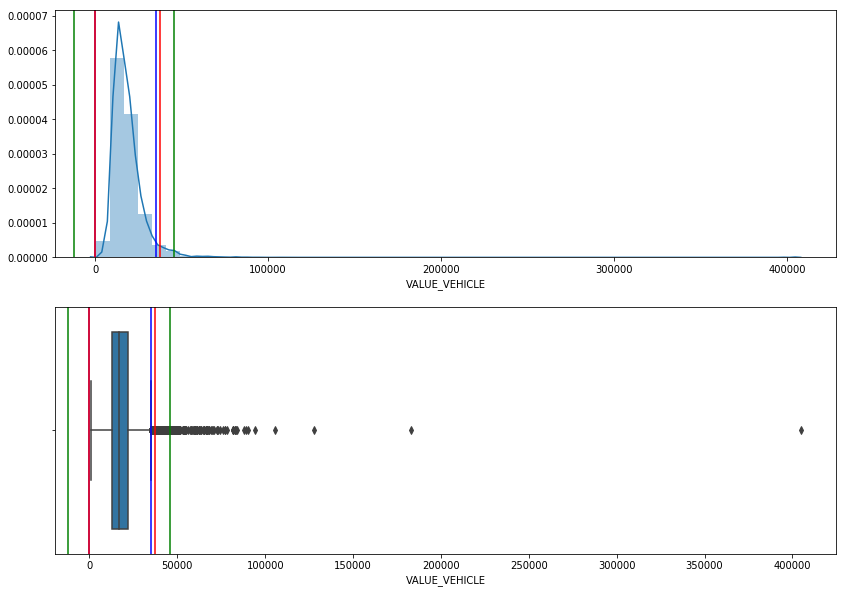Suppose the first thing we'll do is cut the cars valuated over 100K UDS:

In :
df = df[df['VALUE_VEHICLE'] < 100_000]

In :
fig, axs = plt.subplots(2, 1, sharex=True, figsize=(14,10))

plt.subplot(211)
sns.distplot(df['VALUE_VEHICLE'])
plt.axvline(x=min_iqr, color='blue')
plt.axvline(x=max_iqr, color='blue')
plt.axvline(x=min_mean, color='red')
plt.axvline(x=max_mean, color='red')
plt.axvline(x=min_median, color='green')
plt.axvline(x=max_median, color='green')

plt.subplot(212)
sns.boxplot(df['VALUE_VEHICLE'])
plt.axvline(x=min_iqr, color='blue')
plt.axvline(x=max_iqr, color='blue')
plt.axvline(x=min_mean, color='red')
plt.axvline(x=max_mean, color='red')
plt.axvline(x=min_median, color='green')
plt.axvline(x=max_median, color='green')

Out:
<matplotlib.lines.Line2D at 0x7f763e0f6160>